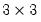Command Reference : Matrix Language Reference

 @inner
Inner product of vectors, series, matrices, group.
Syntax: @inner(v1, v2[, s])
v1: vector, series
v2: vector, series
s: (optional) sample object, string
Return: scalar
If used with two vectors, v1 and v2, @inner returns the dot or inner product of the two vectors.
If used with two series, v1 and v2, @inner returns the dot or inner product of the two series for the workfile or optional sample. If used with two series, @inner returns the inner product of the series using observations in the workfile sample, or optional sample. Observations with NAs will be skipped.
Syntax: @inner(o[, s])
o: matrix, group
s: (optional) sample object, string
Return: sym
If used with a matrix or sym, o, @inner returns the moment matrix, oo. Each element of the result is the vector inner product of two columns of the source matrix. The size of the resulting sym is the number of columns in o.
If used with a group, @inner returns the uncentered second moment matrix of the data in the group, g, using the observations in the sample, smp. If no sample is provided, the workfile sample is used. Observations with NAs will be skipped
Examples
series s1 = nrnd
series s2 = nrnd
scalar sc1 = @inner(s1, s2)
scalar sc2 = @inner(s1, s2, "1990q1 2022q3")
computes the inner product of the series for the workfiles sample, in SC1, and for the sample 1990q1 to 2022q3 in SC2.
vector v1 = @fill(1, 2, 3)
vector v2 = @fill(5, 6, 7)
scalar sc1 = @inner(v1, v2)
computes the inner product of V1 and V2.
scalar sc1 = @inner(v1)
sym sym1 = @inner(v1.@t)
produces a scalar inner product for V1, and thesym matrix from the matrix multiple for SYM1.
sample smp1 "1990q1 2022q3"
group gr1 x y z
sym sym2 = @inner(gr1)
sym sym3 = @inner(gr1, smp1)
computes the matrix multiplications with the data in the groups GR1, producingsymmetric matrices. SYM2 is based on computations using the data in the workfile sample and SYM3 uses the sample “1990q1 2022q3”.
Cross-references